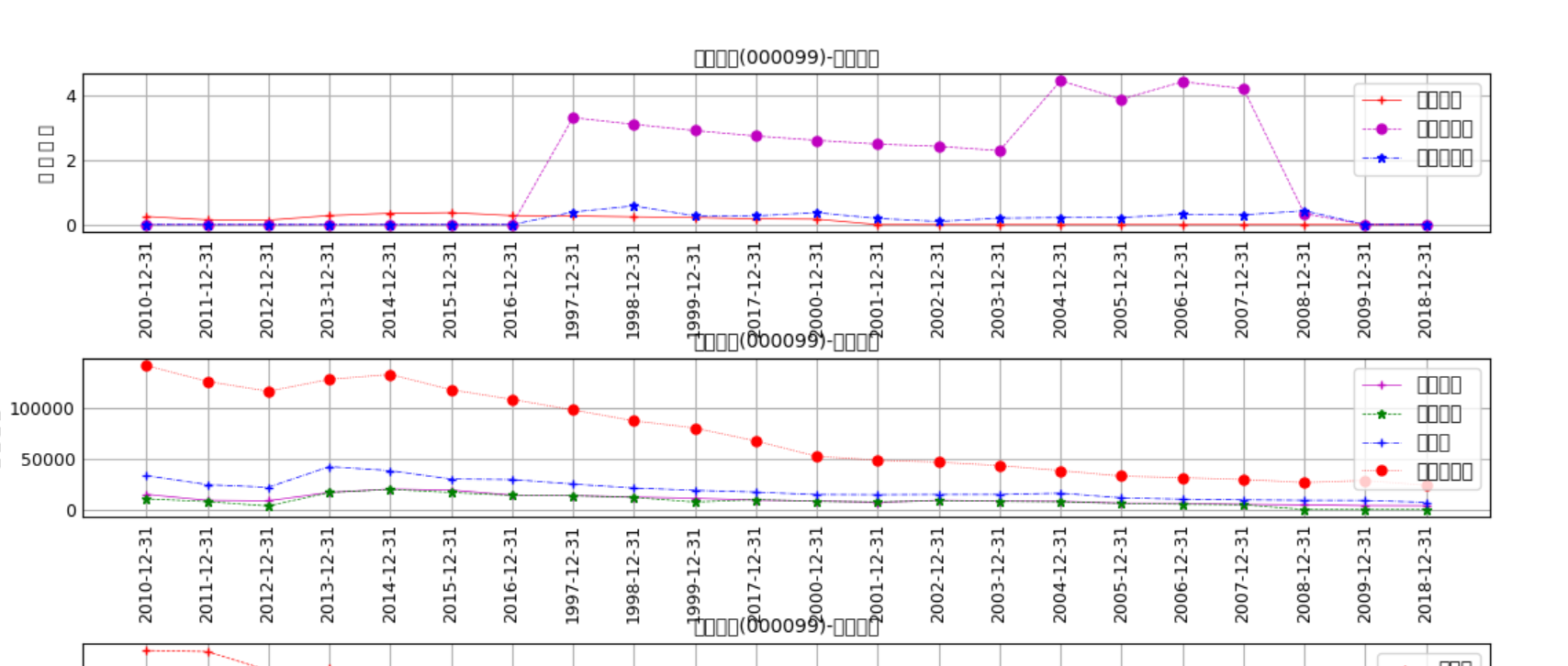python matplotlib作图时，提示缺少字体去哪找字体？D:\Python37\lib\site-packages\matplotlib\backends\backend_agg.py:211: RuntimeWarning: Glyph 24635 missing from current font.
font.set_text(s, 0.0, flags=flags)

D:\Python37\lib\site-packages\matplotlib\backends\backend_agg.py:180: RuntimeWarning: Glyph 24635 missing from current font.
font.set_text(s, 0, flags=flags)

字体无法正常显示 请问怎样修改？


2个回答

pl.rcParams['font.sans-serif']=['SimHei'] #显示中文标签
pl.rcParams['font.serif'] = ['KaiTi']
pl.rcParams['axes.unicode_minus'] = Falsepython matplotlib.pyplot画折线图

vs中使用python操作matplotlib时发生错误

python matplotlib 画两条折线图 其中一组数据有空值 如何处理？

python matplotlib 画两条折线图 其中一组数据有空值 如何处理？ import csv from datetime import datetime from matplotlib import pyplot as plt filename = 'data.csv' with open(filename) as f: reader = csv.reader(f) header_row = next(reader) dates = [] temp1 = [] temp2 = [] for row in reader: time = row + '-' + row + '-' + row current_date = datetime.strptime(time, "%Y-%m-%d") dates.append(current_date) tempm1 = int(float(row)) temp1.append(tempm1) tempm2 = int(row) temp2.append(tempm2) fig = plt.figure(dpi=128, figsize=(10,6)) plt.plot(dates, temp1, c='red') plt.plot(dates, temp2, c='blue') plt.show() 报错： C:\Users\yo\AppData\Local\Programs\Python\Python37\python.exe F:/论文/data/compare/compare.py Traceback (most recent call last): File "F:/论文/data/compare/compare.py", line 26, in <module> tempm2 = int(row) ValueError: invalid literal for int() with base 10: '20.075' Process finished with exit code 1 试过if 不为空值处理，然后数据对不齐了 我想要如果是空值图上就不显示 数据是有小数的所以就用int float 处理 请各位大神帮忙 谢谢！

python matplotlib显示单色图问题

 def initArray(num): img = np.zeros((256,256)) for x in range(0,256): for y in range(0,256): img[x][y] = num return img fig = plt.figure() ax = fig.add_subplot() ax.imshow(initArray(20),cmap='gray') plt.show()  按说initArray的参数变化，显示的图片应该是不同的单色图，可是显示显示的全是黑色怎么回事？

python安装matplotlib报错

python用matplotlib画K线

import matplotlib.pyplot as plt import matplotlib.finance as mpf with open('SH#600004.txt') as obj: text=obj.readlines() baseinfo=text dayinfo=text[2:-1] date_list =[dayinfo[i].split(',') for i in range(len(dayinfo))] open_list =[dayinfo[i].split(',') for i in range(len(dayinfo))] high_list =[dayinfo[i].split(',') for i in range(len(dayinfo))] low_list =[dayinfo[i].split(',') for i in range(len(dayinfo))] close_list=[dayinfo[i].split(',') for i in range(len(dayinfo))] quotes=zip(date_list,open_list,high_list,low_list,close_list) #N=100 #open_list[:N],high_list[:N],low_list[:N],close_list[:N] fig,ax=plt.subplots() mpf.candlestick_ohlc(ax,quotes,width=0.6,colorup='r',colordown='green') plt.title(baseinfo) plt.xlabel(date_list) plt.rcParams['font.sans-serif']=['SimHei'] #用来正常显示中文标签 plt.rcParams['axes.unicode_minus']=False #用来正常显示负号 plt.show() **#以下是报错信息 C:\ProgramData\Anaconda3\python.exe G:/python/test/test.py C:\ProgramData\Anaconda3\lib\site-packages\matplotlib\cbook\deprecation.py:106: MatplotlibDeprecationWarning: The finance module has been deprecated in mpl 2.0 and will be removed in mpl 2.2. Please use the module mpl_finance instead. warnings.warn(message, mplDeprecation, stacklevel=1) Traceback (most recent call last): File "G:/python/test/test.py", line 18, in <module> mpf.candlestick_ohlc(ax,quotes,width=0.6,colorup='r',colordown='green') File "C:\ProgramData\Anaconda3\lib\site-packages\matplotlib\finance.py", line 737, in candlestick_ohlc alpha=alpha, ochl=False) File "C:\ProgramData\Anaconda3\lib\site-packages\matplotlib\finance.py", line 794, in _candlestick height = open - close TypeError: unsupported operand type(s) for -: 'str' and 'str'** 我搜了下知道日期格式转换需要用到date2num这个函数 我的文本里面日期格式是XXXX/XX/XX的形式 请问quotes一项里日期需求的格式是什么?

python matplotlib报错 mac系统，急！！！

python Matplotlib 怎么把两个箱图分开，但要求在同一图上

python3.8下载matplotlib模块时，总是出现以下错误 ERROR: Command errored out with exit status 1: 'c:\users\air\python\python38\python.exe' -u -c 'import sys, setuptools, tokenize; sys.argv = '"'"'C:\\Users\\air\\AppData\\Local\\Temp\\pip-install-5ntug3if\\matplotlib\\setup.py'"'"'; __file__='"'"'C:\\Users\\air\\AppData\\Local\\Temp\\pip-install-5ntug3if\\matplotlib\\setup.py'"'"';f=getattr(tokenize, '"'"'open'"'"', open)(__file__);code=f.read().replace('"'"'\r\n'"'"', '"'"'\n'"'"');f.close();exec(compile(code, __file__, '"'"'exec'"'"'))' install --record 'C:\Users\air\AppData\Local\Temp\pip-record-rp63qqyg\install-record.txt' --single-version-externally-managed --compile Check the logs for full command output. ![图片说明](https://img-ask.csdn.net/upload/201911/20/1574238491_785213.png) 有那位大神可以帮我看一下？

python用matplotlib画图时如何使坐标轴的数字显示为千分位的形式如100,000,000

python用matplotlib画图时如何使坐标轴的数字显示为千分位的形式如100,000,000

Python使用matplotlib绘图怎么把图的标题放到图下方

 plt.title('助攻表现（常规赛）') plt.ylabel('场均助攻数') plt.xlabel('年份-冠军球队') plt.ylim(17) plt.setp(plt.xticks(idx,tick),rotation=90) plt.legend(bbox_to_anchor=(1.15,1)) plt.show()  怎么修改呢，像R那样把标题放在图下面

python import matplotlib.pyplot 报错 DLL load failed

Anaconda3.5 python3.6 pycharm win7系统 import matplotlib没问题 matplotlib 2.1.0 import matplotlib.pyplot 报错 ![图片说明](https://img-ask.csdn.net/upload/201712/07/1512653783_182335.png) 另外，import matplotlib之前有个错误，更新了numpy之后好了。 在网上没找到类似的问题

matplotlib所绘制的图像在全屏查看时比例失调，怎么解决？

Java基础知识面试题（2020最新版）

String s = new String(" a ") 到底产生几个对象？

Linux面试题（2020最新版）

Linux命令学习神器！命令看不懂直接给你解释！

loonggg读完需要3分钟速读仅需 1 分钟大家好，我是你们的校长。我之前讲过，这年头，只要肯动脑，肯行动，程序员凭借自己的技术，赚钱的方式还是有很多种的。仅仅靠在公司出卖自己的劳动时...

85后蒋凡：28岁实现财务自由、34岁成为阿里万亿电商帝国双掌门，他的人生底层逻辑是什么？...

MySQL数据库面试题（2020最新版）

《经典算法案例》01-08：如何使用质数设计扫雷（Minesweeper）游戏